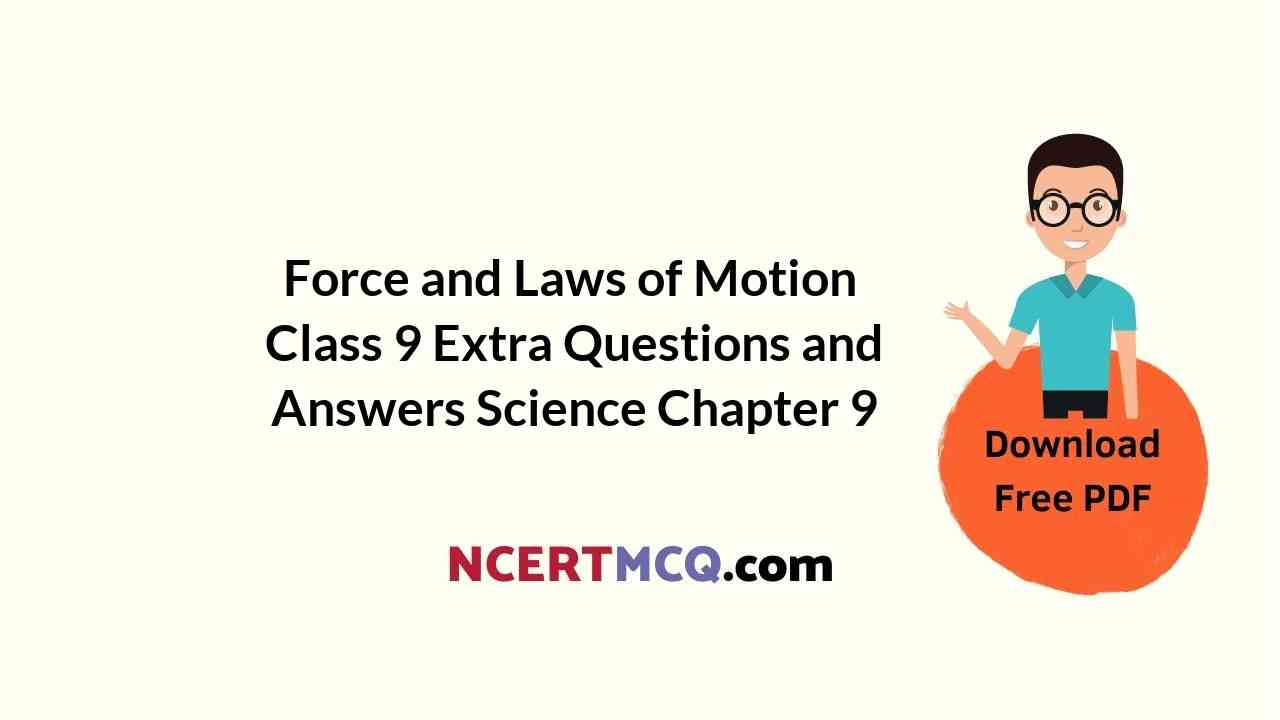In this page, we are providing Force and Laws of Motion Class 9 Extra Questions and Answers Science Chapter 9 pdf download. NCERT Extra Questions for Class 9 Science Chapter 9 Force and Laws of Motion with Answers will help to score more marks in your CBSE Board Exams. https://ncertmcq.com/extra-questions-for-class-9-science/

Class 9 Science Chapter 9 Extra Questions and Answers Force and Laws of Motion

Extra Questions for Class 9 Science Chapter 9 Force and Laws of Motion with Answers Solutions

Force and Laws of Motion Class 9 Extra Questions Very Short Answer Type

Force And Laws Of Motion Class 9 Numericals Extra Questions Question 1.
Define the following terms

1. Force
2. Balanced forces
3. Unbalanced forces
4. Inertia
5. Momentum
6. Conservation of momentum.

1. Force: It is an entity which when applied on a body changes or tends to change its state of motion and shape.
2. Balanced forces: When a number of forces acting simultaneously on a body do not bring about any change in the state of rest or of uniform motion along a straight line, then the forces acting on the body are said to be balanced.
3. Unbalanced forces: When a number of forces acting simultaneously on a body bring about a change in its state of rest or of uniform motion along a straight line, then these forces acting on the body are said to be unbalanced forces.
4. Inertia: Inertia is the natural tendency of an object to resist a change in its state of motion or rest.
5. Momentum: Momentum of a body is the product of its mass and velocity.
6. Conservation of momentum: In an isolated system, the total momentum remains conserved.

Force And Laws Of Motion Class 9 Extra Questions Question 2.
Name the physical quantity that measures inertia.
Mass of body measures its inertia.

Force And Laws Of Motion Extra Questions Numericals Question 3.
There are three solids made up of aluminum, steel, and wood, of the same shape and same volume. Which of them would have the highest inertia?
Steel ball would have the highest inertia as steel is denser than the other two.

Force And Laws Of Motion Class 9 Extra Numericals Question 4.
Name the factors on which the momentum of a body depends.

• Mass and
• The velocity of the body.

Class 9 Force And Laws Of Motion Extra Questions Question 5.
Define one newton of force.
Force is said to be 1 newton if it produces an acceleration of 1 rn/s2 in a body of 1 kg.

Force And Laws Of Motion Extra Questions Question 6.
An object is thrown vertically upwards. What is its momentum at the highest point?
Since the velocity at the highest point is zero, so the momentum of the object is zero at the highest point.

Extra Numericals Of Force And Laws Of Motion Class 9 Question 7.
State action and reaction when a bullet is fired from the gun.
Action: Force exerted by a spring on the bullet.
Reaction: Force exerted on the gun.

Class 9 Science Chapter 9 Extra Questions And Answers Question 8.
An athlete runs some distance before taking a jump. Why?
To gain momentum so that he may jump higher.

Class 9 Physics Chapter 2 Extra Questions Question 9.
Mention any two effects of force.

• It changes the state of rest or motion of a body.
• It changes the shape of the body.

Class 9 Force And Laws Of Motion Extra Numericals Question 10.
What happens to the momentum of a body whose velocity is halved?
The momentum of the body becomes half.

Class 9 Science Ch 9 Extra Questions Question 11.
Give the magnitude and the direction of the net force acting on the cork of mass 10 g floating on water.
Zero.

Class 9 Science Chapter 9 Extra Numericals Question 12.
A car of mass 1000 kg is moving with velocity 5 m/s. Calculate the momentum of the car.
Given, mass, m = 1000 kg
Velocity, u = 5 m/s
Momentum, P = mυ
P= 1000 x 5 = 5000kg m/s
P= 5000 kg m/s

Class 9 Physics Force And Laws Of Motion Extra Questions Question 13.
A meteorite burns in the atmosphere before it reaches the Earth’s surface. What happens to the linear momentum?
Meteorite burns due to heat produced by frictional force. The linear momentum of the meteorite decreases as a frictional force acts on it.

Class 9th Science Chapter 9 Extra Questions Question 14.
Find the acceleration produced by a force of 2000 N acting on a car of mass 800 kg.
Given,
Mass, m =800kg
Force, F = 2000 N
Using F = ma
a = $$\frac{F}{m}$$
a = $$\frac{2000}{800}$$ = 2.5 m/s2

Force and Laws of Motion Class 9 Extra Questions Short Answer Type 1

Question 1.
Write down SI unit of (i) force (ii) momentum.

• newton (N)
• kg rn/s.

Question 2.
When a person hits a stone, his foot is injured. Why?
When a person hits a stone, the stone exerts an equal force on his foot. Due to this force, his foot gets injured.

Question 3.
Why no force is required to move an object with a constant velocity?
We know, F = ma
When, velocity is constant, then acceleration, a = 0. Hence, F = 0.

Question 4.
Why is it easier to catch a table tennis ball than a cricket ball, even both are moving with the same velocity?
It is easier to catch a table tennis ball because the table tennis ball has less mass (inertia).

Question 5.
Write down the expression for the recoil velocity of the gun.
Recoil velocity of gun is given by,
$$\mathrm{V}_{\mathrm{G}}=\frac{m_{b} v_{b}}{\mathrm{M}_{\mathrm{G}}}$$
mb = mass of bullet
mG = mass of gun
υb = velocity of bullet
VG = recoil velocity of gun.

Force and Laws of Motion Class 9 Extra Questions Numericals

Question 6.
A car of mass 1000 kg moving with a velocity of 54 km/h hits a wall and comes to rest in 5 seconds. Find the force exerted by the car on the wall.
Given, Mass = 1000 kg
Time = 5 s
Initial velocity, u = 54 km/h = 15 m/s
Final velocity, υ = 0 m/s
Time, t = 5s
using, F = m a
F = m$$\left(\frac{v-u}{t}\right)$$ = 1000 $$\left(\frac{0-15}{5}\right)$$ = -3000N

Question 7.
A body of mass 100g is at rest on a smooth surface. A force of 0.1-newton act on it for 5 seconds. Calculate the distance traveled by the body.
Given, Mass of body = 100 g = 0.1 kg
Force, F = 0.1N
Time, t = 5s
Initial velocity, u = 0 m/s
Using formula, F = ma
⇒ a = $$\frac{F}{m}=\frac{0.1}{0.1}$$ = 1 m/s2

Using formula, s = ut + at2
s = ut + $$\frac {1}{2}$$at2
⇒ s = 0 + $$\frac {1}{2}$$ x 1 x (5)2
∴ s = 12.5 m

Question 8.
A bullet of mass 200 g is fired from a gun of mass 10 kg with a velocity of 100 m/s. Calculate the velocity of recoil.
Given, Mass of bullet, m = 200 g = 0.2 kg
Massofgun, M = 10 kg
Velocity of bullet, Vb = 100 m/.s
Recoil velocity of gun, VG = $$\frac{m v_{b}}{\mathrm{M}}$$
VG = – $$\frac{0.2 \times 100}{10}$$ = 2m/s
Recoil velocity of gun, VG = 2 m/s

Question 9.
Two spheres of masses 20 g and 40 g moving in a straight line in the same direction with velocities of 3 mIs and 2 m/s respectively. They collide with each other and after the collision, the sphere of mass 20 g moves with a velocity of 2.5 miles. Find the velocity of the second ball after confusion.
Given, m1 = 20 g = 20 x 10-3 kg
m1 = 40g = 40g x 10-3kg
u1 = 3 m/s
u2 = 2 m/s
υ1 = 2.5 m/s
Applying conservation of linear momentum,
m1u1 + m2u2 = m1υ1 + m2υ2
20 x 10-3 x 3 + 40 x 10-3 x 2 = 20 x 10-3 x 2.5 + 40 x 10-3 x υ2
υ2 = 2.25m/s.

Force and Laws of Motion Class 9 Extra Questions Short Answer Type 2

Question 1.
Newton’s first, second, and third law of motion.
Newton’s first law of motion: An object remains in a state of rest or of uniform motion along a straight line unless compelled to change that state by an applied force.

Newton’s second law of motion: The second law of motion states that the rate of change of momentum of an object is proportional to the applied unbalanced force in the direction of the force.

Newton’s third law of motion: According to the third law of motion to every action, there is an equal opposite reaction and they act on two different bodies.

Question 2.
What is momentum? Write its SI unit. Interpret force in terms of momentum. Represent the following graphically.
(a) momentum versus velocity when mass is fixed.
(b) momentum versus mass when velocity is constant.
Momentum gives an idea about the quantity of motion contained in a body.
The momentum of (P) of an object is defined as the product of its mass (m) and velocity (υ).
P = mυ
SI unit of momentum is kg ms-1
Graphs
(a) If m = constant
P ∝ υ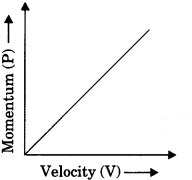(b) If v = constant
P ∝ m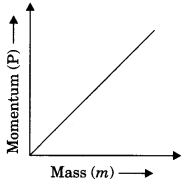Question 3.
“Action and reaction are equal and opposite but even they do not cancel each other.” Explain, why?
Two equal and opposite forces can cancel each other if they act on the same body. But action and reaction do not act on the same body. They act on two different bodies. Hence cannot cancel each other.

Question 4.
Why are the wheels of vehicles provided with mudguards?
The rotating wheels of a vehicle throw out mud sticking to it tangentially due to the inertia of direction. The mudguards stop this mud to fall on another vehicle just moving behind the vehicle.

Question 5.
A car weighing 1600 kg moving with a velocity of 30 mIs retards uniformly coming to rest in 20 seconds. Calculate the
1. Initial and final momentum of the car.
2. Rate of change of linear momentum of the car.
3. Acceleration of the car.
Given, mass, m = 1600 kg
Initial velocity, u = 30 m/s
Final velocity, υ = 0 m/s
Time, t = 20s
1. Initial momentum, Pi = mu = 1600 x 30
Pi = 4800 kg m/s
Final momentum, Pf = mυ = 1600 x 0
Pf = 0 kg m/s

2. Rate of change of linear momentum
= $$\frac{P_{f}-P_{i}}{t}$$ = $$\frac{0-4800}{20}$$ = – 240N
a = $$\frac{v-u}{t}$$

3.  Acceleration,
$$\frac{0 – 30}{20}$$
a = – 1.5 m/s2.

Question 6.
Using the second law of motion, derive the relation between force and acceleration. A bullet of 10 g strikes a sand-bag at a speed of 103 ms2 and gets embedded after traveling 5 cm. Calculate
1. the resistive force exerted by the sand on the bullet.
2. the time is taken by the bullet to come to rest.
According to the second law of motion,
The rate of change of momentum is directly proportional to the force applied.
F ∝ $$\frac{m(v-u)}{t}$$
F = $$\frac{km(v-u)}{t}$$
∴ F = kma
Take k = 1 in SI system
∴ F = ma

1. Given, mass of bullet, m = 10g = 10 x 10-3kg
Initial velocity of the bullet, u = 103m/s
Distance travelled by the bullet, s = 5 cm = 5 x 10-2 m
Final velocity of the bullet = 0
Using the formula, υ-2 – u-2 = 2as
⇒ 0 – (103)2 = 2 x a x 5 x 10-2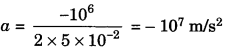f = m x a = 10 x 10-3 x (107) = – 105 N

2. From the formula,
υ = u + at
⇒  t = $$\frac {υ – u}{a}$$ = $$\frac{0-10^{3}}{-10^{7}}$$ = 10-4 s = 0.0001 s

Question 7.
An object is placed on a rough surface. The external force of 20 N is acting on the body and 10 N frictional force is acting on the body.
Find
1. The net force on an object
2. Acceleration of an object if the mass of an object is 2 kg
3. The velocity of the object after 2 seconds if the object is started from rest.
Given,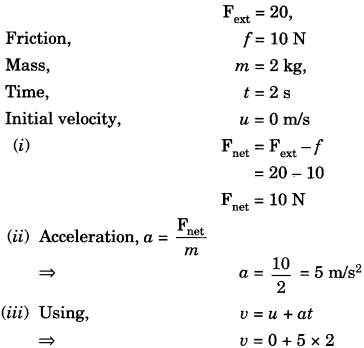∴ υ = 10 m/s

Force and Laws of Motion Class 9 Extra Questions Long Answer Type

Question 1.
Explain why?
(a) A cricket player lowers his hands while catching the ball.
(b) The vehicles are fitted with shockers.
(c) A karate player breaks the pile of tiles or bricks with a single blow.
(d) In a high jump athletic event, the athletes are allowed to fall either on a sand bed or cushioned bed.
(e) In a moving car, the drivers and other passengers are advised to wear seat belts.
(f) China and glassware are packed with soft materials.
(g) Athletes are advised to come to a stop slowly after finishing a fast race.
(a) if a player does not lower his hands while catching the ball, the time to stop the ball is very small. So a large force has to be applied to reduce the velocity of the ball to zero or to change the momentum of the ball. When a player lowers his hands, the time to stop the ball is increased and hence less force has to be applied to cause the same change in the momentum of the ball. Therefore, the hands of the player are not injured.

(b) The vehicles are fitted with shockers (i.e., springs)
The floor of a vehicle is cónnected to the lower part of the vehicle by springs or shockers. When the vehicle moves over a rough road, the force due to jerks is transmitted to the floor of the vehicle through the shockers. The shockers increase the time of transmission of the force of jerk to reach the floor of the vehicle. Hence less force is experienced by the passengers in the vehicles.

(c) A karate player can break a pile of tiles with a single blow of his hand because he strikes the pile of tiles with his hand very fast, during which the entire linear momentum of the fast-moving hand is reduced to zero in a very short interval of time. This exerts a very large force on the pile of tiles which is sufficient to break them, by a single blow of his hand.

(d) In a high jump athletic event., the athletes are allowed to fall either on a sand bed or cushioned bed: This is done to increase the time of athletes fall to stop after making the high jump, which decreases the rate of change of linear momentum and decreases the impact.

(e) In a moving car, the drivers and other passengers are advised to wear seat belts: When brakes are applied sudden1y, the passengers of the car fall forward due to the inertia of motion. The seat belt worn by passengers of the car prevents them from falling forward suddenly. This enables the entire linear momentum of the passengers to reduce to zero over a long interval of time, hence it prevents injury.

(f) China and glassware are packed with soft material: China and glassware are wrapped in paper before packing to avoid breakage while transporting. During transportation, there may be collisions due to ta jerks of the packed wares. Soft material like paper slows down their rate of change of linear momentum. As a result, the impact is reduced and items are not broken.

(g) Athletes are advised to come to stop slowly after finishing a fast race: By doing so, he decreases the rate of change of linear momentum by increasing the time interval and hence, reducing the impact, which reduces injury.

Question 2.
Explain
(a) How do we swim?
(b) Why does a gun recoil?
(c) It is difficult to walk on sand or ice.
(d) The motion of rocket.
(e) Why does a fireman struggle to hold a hose-pipe?
(f) Rowing of a boat.
(g) When a man jumps out from a boat, the boat moves backward.
(h) Walking of a person.
(a) While swimming, a swimmer pushes the water backward with his hands (i.e., he applies force in the backward direction, which is known as action.) The reaction offered by the water to the swimmer pushes him forward.

(b) Recoiling of a gun: When a bullet is fired from a gun, it exerts a forward force on the bullet and the bullet exerts an equal (in magnitude) and opposite (in direction) force on the gun. Due to the high mass of the gun, it moves a little distance backward and gives a backward jerk to the shoulder of the gunman.

(c) It is difficult to walk on sand or ice: When our feet press the sandy ground in the backward direction, the sand gets pushed away and as a result, we get only a small reaction (forward) from the sandy ground making it difficult to walk.

(d) Rocket propulsion Before firing the rocket, the total linear momentum of the system is zero because the rocket is in the state of rest. When it is fired, chemical fuels inside the rocket are burnt and the hot gases are passed through a nozzle with great speed. According to the law of conservation of linear momentum, the total linear momentum after firing must be equal to zero. As the hot gases gain linear momentum to the rear on leaving the rocket, the rocket acquires equal linear momentum in the upward i.e., opposite direction.

(c) A fireman has to make a great effort to hold a hose-pipe to throw a stream of water on the fire to extinguish it. This is because the stream of water rushing through the hose-pipe in the forward direction with a large speed exerts a large force on the hose-pipe in the backward direction which is known as the reaction force. This reaction force tends to move the hose-pipe in the backward direction. Therefore, a fireman struggles to hold the hose-pipe strongly to keep it at rest.

(f) Rowing of a boat: The boatman during the rowing of a boat pushes the water backward with oars (this is an action of the boatman). According to the third law of motion, water exerts an equal (in magnitude) and opposite (in direction) push on the boat which moves it forward (this is a reaction by water). Thus, harder the boatman pushes back the water with oars (i.e., greater is the action), greater is the reaction force exerted by water, and faster the boat moves in the forward direction.

(g) When a man jumps out from a boat, the boat moves backward: When the passengers start jumping out of a rowing boat, they push the boat backward with their feet. The boat exerts an equal (in magnitude) and opposite (in direction) force on passengers in the forward direction which enables them to move forward.

(h) Walking of a person: When a person walks on the ground, he pushes the ground with his foot in the backward direction by pressing the ground. This push is known as the action, According to Newton’s third law of motion, an equal and opposite reaction acts on the foot of the person by the ground. This reaction (force) of the ground on the person pushes him forward.

Force and Laws of Motion Class 9 Extra Questions HOTS

Question 1.
Two balls of the same size but of different materials, rubber and iron are kept on the smooth floor of a moving train. The brakes are applied suddenly to stop the train. Will the balls start rolling? If so, in which direction? Will they move with the same speed? Give reasons for your answer
Yes, both the balls will start rolling in the direction opposite to the motion of the train. The speed of two balls will be different as the inertia of the two balls are different.

Question 2.
Two identical bullets are fired on by a light rifle and another by a heavy rifle with the same force. Which rifle will hurt the shoulders more and why?
The light rifles will hurt more as the recoil velocity of the light rifle will be greater.

Question 3.
Velocity versus time graph of a ball of mass 50 g rolling on a concrete floor is shown in the figure. Calculate the acceleration and frictional force of the floor on the ball.Given, Mass of ball, m = 50 g = 50 x 10-3 kg
Acceleration can be calculated by υ – t graph,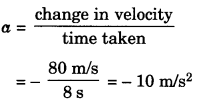= $$\frac{80 \mathrm{m} / \mathrm{s}}{8 \mathrm{s}}$$ = – 10 m/s2
a = – 10 m/s2
Friction force, F = ma
F = 50 x 10-3 x 10
F = 0.5 N

Question 4.
A truck of mass M is moved under a force F. if the truck is then loaded with an object equal to the mass of the truck and the driving force is halved, then how does the acceleration change?
Given, Initially
M1 = M and F1 = F
Finally
M2 = 2M and F2=$$\frac {F}{2}$$
Hence, a1 = $$\frac{F_{1}}{M_{1}}$$
a1 = $$\frac{F}{M}$$

and a2 = $$\frac{F_{2}}{M_{2}}$$
a2 = $$\frac{\mathrm{F}}{2 \times 2 \mathrm{M}}$$ = $$\frac{F}{4M}$$
$$\frac{a_{1}}{a_{2}}=\frac{F / M}{F / 4 M}=4$$
a2 = $$\frac{a_{1}}{4}$$
Acceleration will become one fourth.

Question 5.
Derive the unit of force using the second law of motion. A force of 5 N produces an acceleration of 8 ms-2 on a mass m1 and an acceleration of 24 ms-2 on a mass m2. What acceleration would the same force provide if both the masses are tied together?
Given, Force, F = 5N
a1 = 8 m/s2
and a1 = 24 m/s2
From formula
m1 = $$\frac{F}{a_{1}}$$ = $$\frac{5}{8}$$ kg
and m2 = $$\frac{F}{a_{2}}$$ = $$\frac{5}{24}$$ kg
When both the masses tied together
a =$$\frac{\mathrm{F}}{m_{1}+m_{2}}$$
a = $$\frac{5}{\frac{5}{8}+\frac{5}{24}}$$ = 6m/s2
a = 6m/s2

Force and Laws of Motion Class 9 Extra Questions Value Based (VBQs)

Question 1.
During a cricket match, a new player Ayush injured his hand while catching the ball. His friend Rudra advised him to catch the ball by lowering his hands backward. When Ayush got another chance to catch the ball, he successfully caught the ball without injuring his hands.
(a) A cricket player lowers his hands while catching the ball. Explain why.
(b) Write down the values shown by Rudra.
(a) If a player does not lower his hands while catching the ball, the time to stop the ball will be very small. So a large force has to applied to reduce the velocity of the ball to zero or to change the momentum of the ball. When a player lowers his hands, the time to stop the ball is increased and hence less force has to be applied to cause the same change in momentum of the ball. Therefore, the hands of the player are not injured.

(b) Rudra is a good person as he helped his friend Ayush. He is a knowledgeable person.

Question 2.
During servicing his bike Aman advised the mechanic to oil the shockers for its proper functioning.
(a) The vehicles are fitted with shockers. Explain why.
(b) Write down the values shown by Aman.